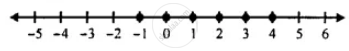# Solve the Inequation 5(X – 2) > 4 (X + 3) – 24 and Represent Its Solution on a Number Line. Given the Replacement Set is {-4, -3, -2, -1, 0, 1, 2, 3, 4}. - Mathematics

Sum

Solve the inequation 5(x – 2) > 4 (x + 3) – 24 and represent its solution on a number line.

Given the replacement set is {-4, -3, -2, -1, 0, 1, 2, 3, 4}.

#### Solution

5(x – 2) > 4 (x + 3) – 24

⇒ 5x - 10 > 4x + 12 - 24

⇒ 5x - 4x > 10 - 12

⇒ x > -2

Since replacement set ={-4, -3, -2, -1, 0, 1, 2, 3, 4}

∴ Solution set = {-1, 0, 1, 2, 3, 4}

∴ Solution set on a number line is shown below.Is there an error in this question or solution?

#### APPEARS IN

Selina Concise Mathematics Class 8 ICSE
Chapter 15 Linear Inequations
Exercise 15 (B) | Q 11 | Page 175